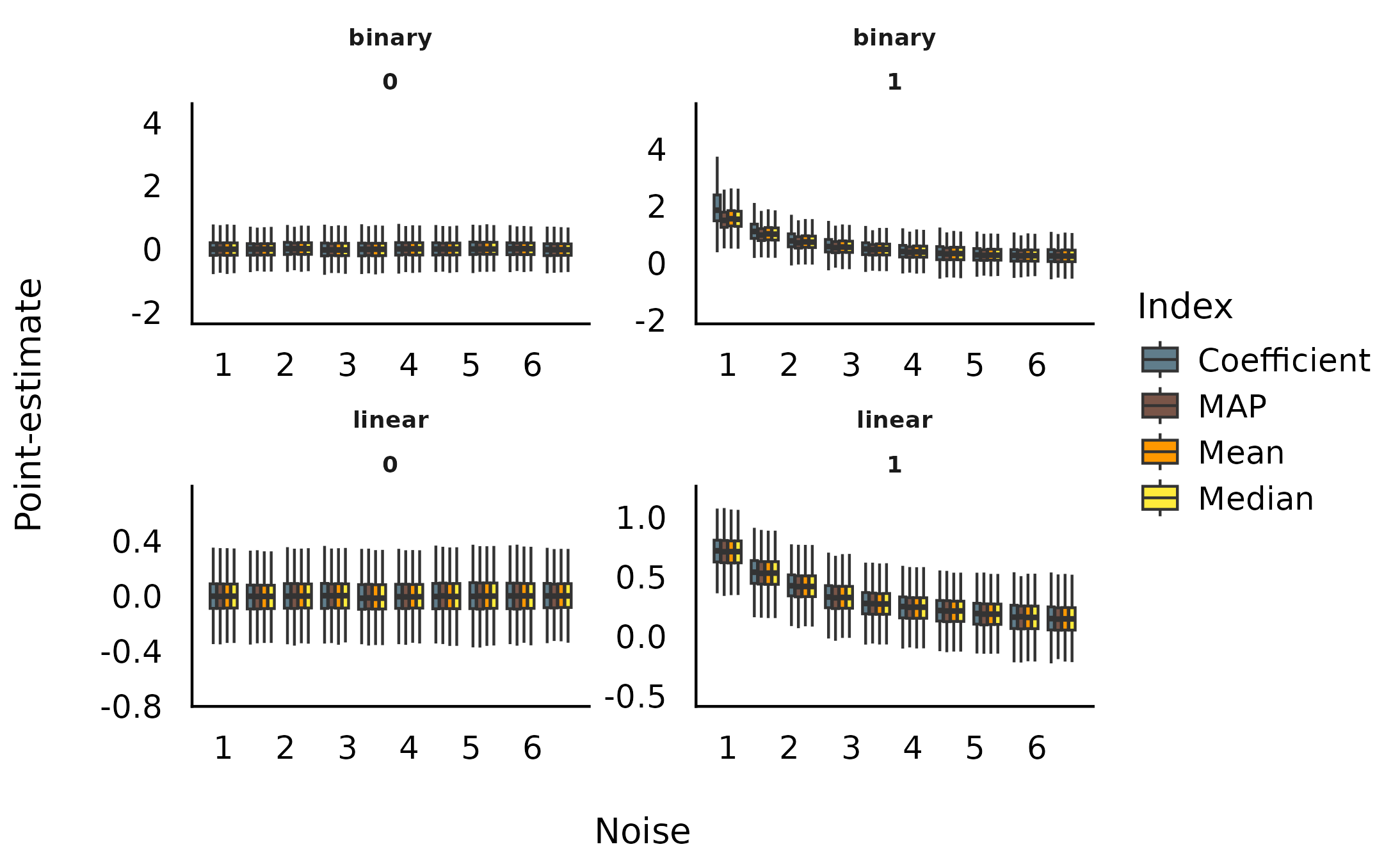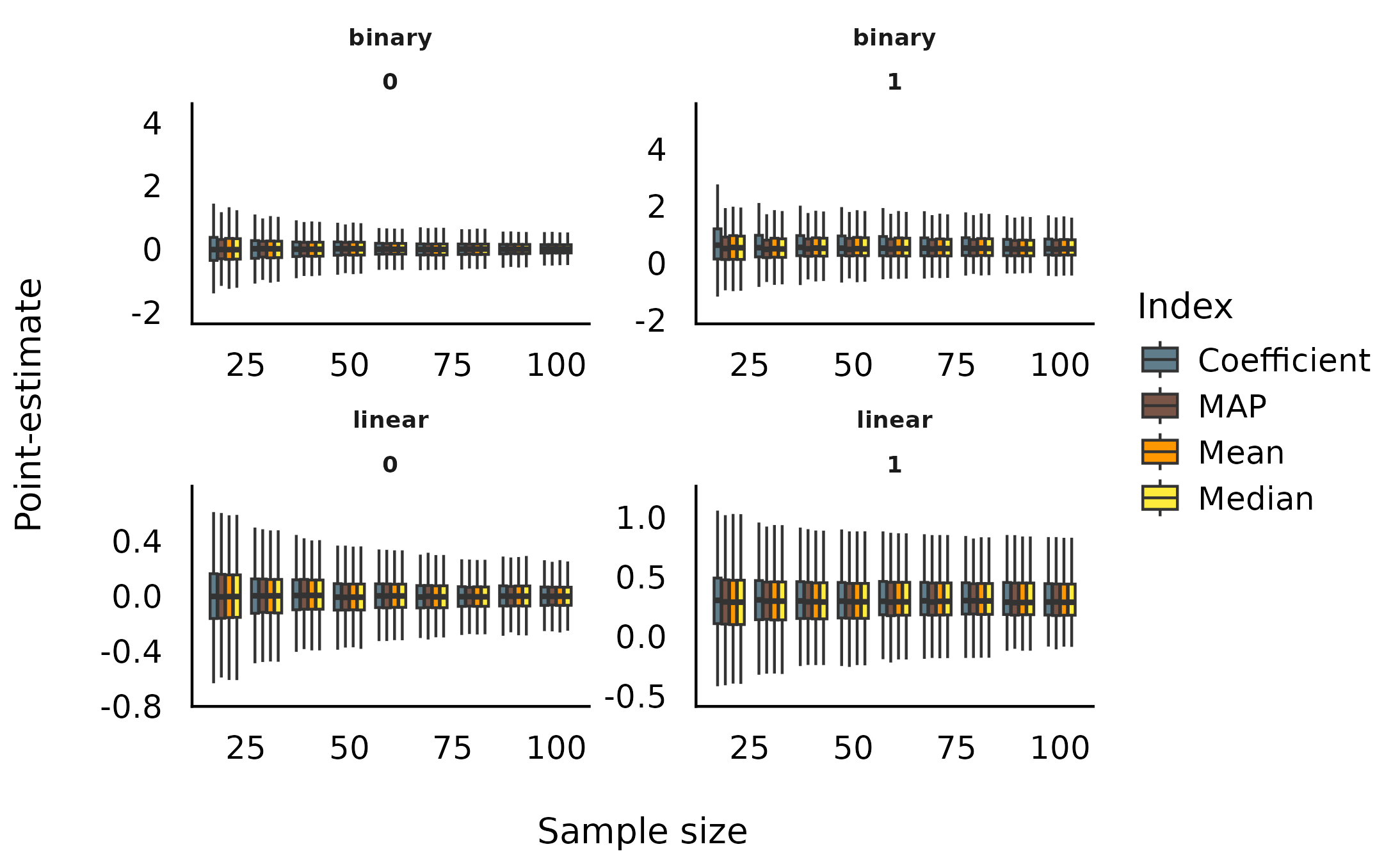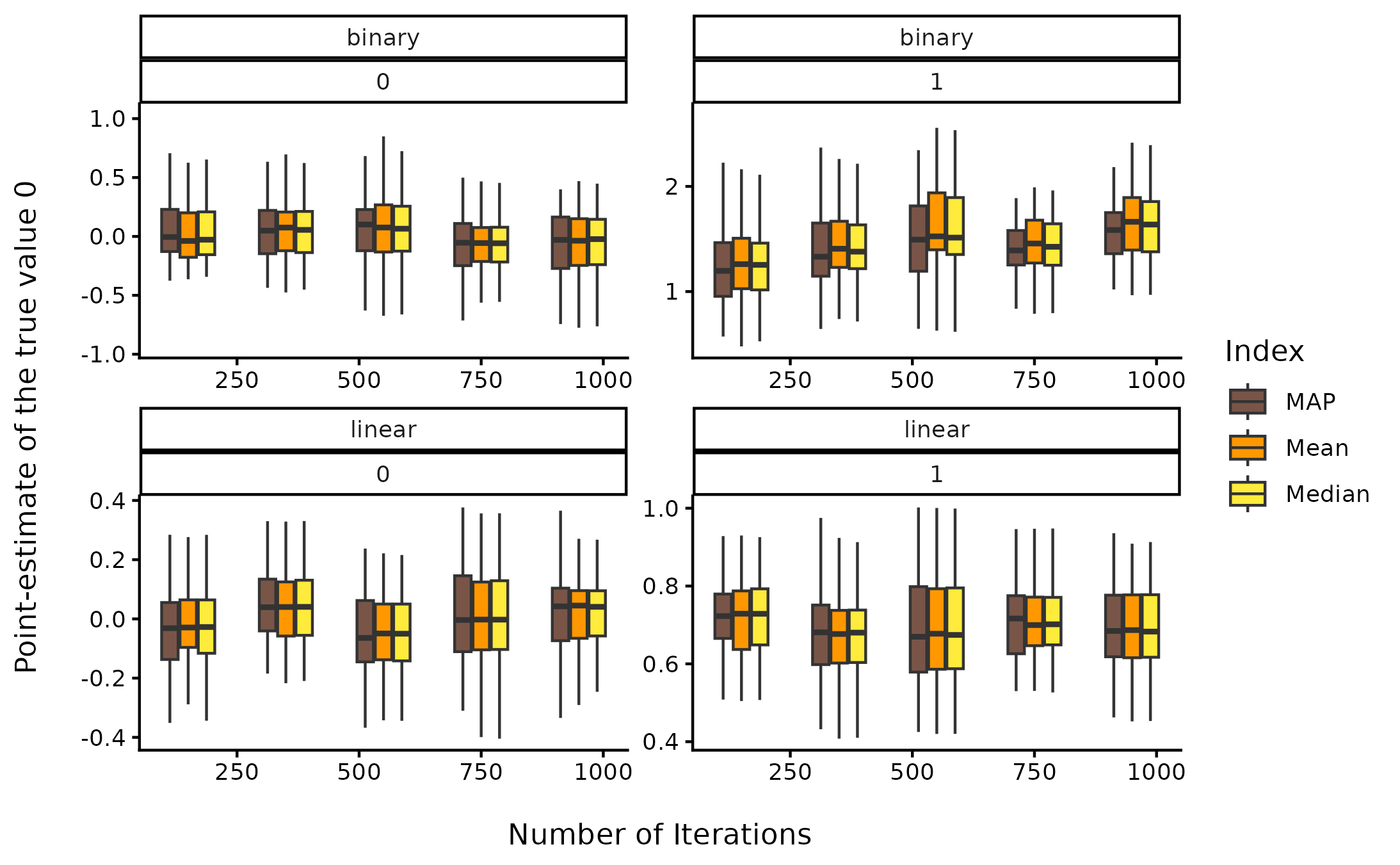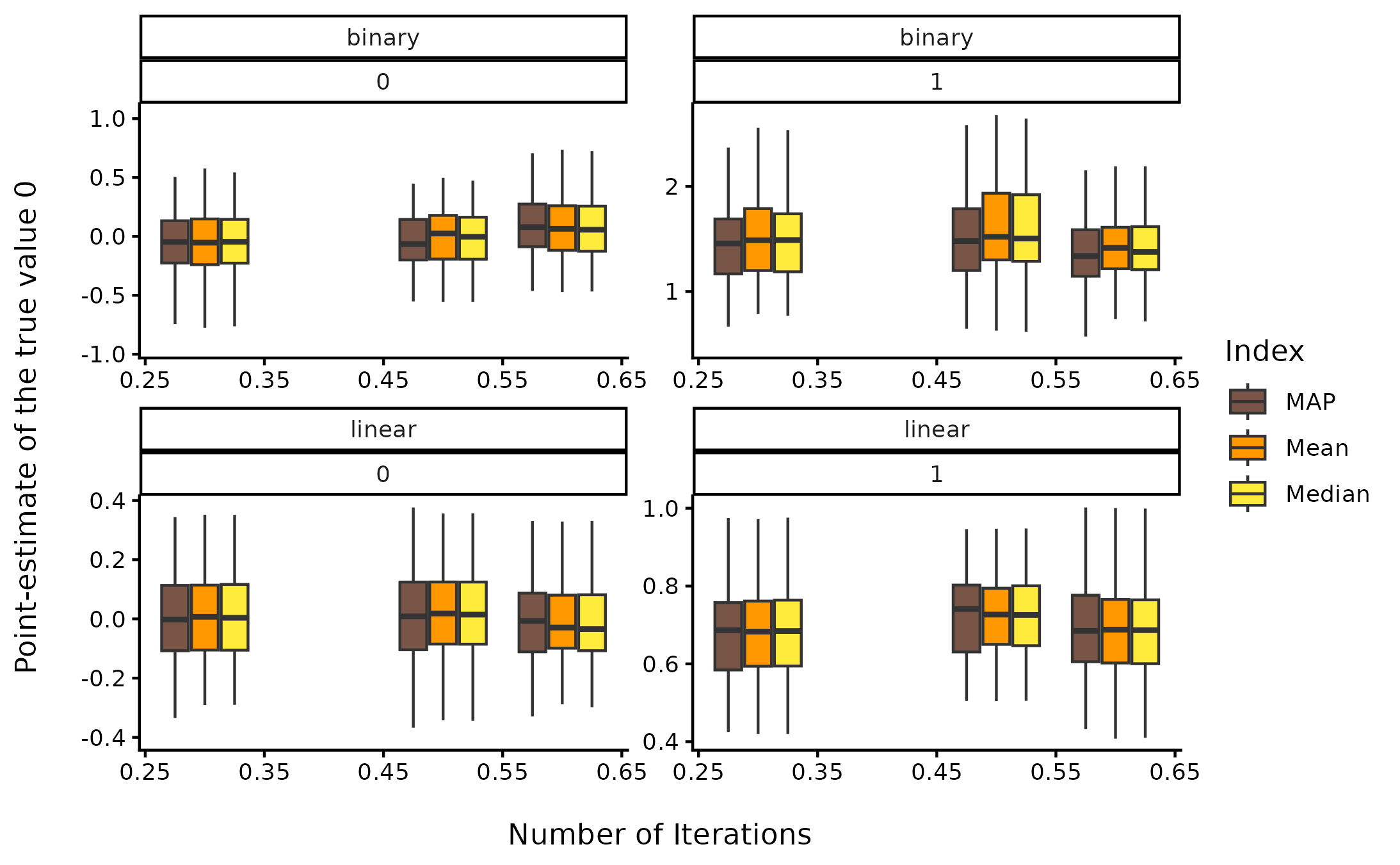This vignette can be referred to by citing the package:

• Makowski, D., Ben-Shachar, M. S., & Lüdecke, D. (2019). bayestestR: Describing Effects and their Uncertainty, Existence and Significance within the Bayesian Framework. Journal of Open Source Software, 4(40), 1541. https://doi.org/10.21105/joss.01541

## Effect Point-Estimates in the Bayesian Framework

### Introduction

One of the main difference between the Bayesian and the frequentist frameworks is that the former returns a probability distribution for each effect (i.e., a model parameter of interest, such as a regression slope) instead of a single value. However, there is still a need and demand - for reporting or use in further analysis - for a single value (point-estimate) that best characterises the underlying posterior distribution.

There are three main indices used in the literature for effect estimation: - the mean - the median - the MAP (Maximum A Posteriori) estimate (roughly corresponding to the mode - the “peak” - of the distribution)

Unfortunately, there is no consensus about which one to use, as no systematic comparison has ever been done.

In the present work, we will compare these three point-estimates of effect with each other, as well as with the widely known beta, extracted from a comparable frequentist model. These comparisons can help us draw bridges and relationships between these two influential statistical frameworks.

### Experiment 1: Relationship with Error (Noise) and Sample Size

#### Methods

We will be carrying out simulation aimed at modulating the following characteristics:

• Model type: linear or logistic.
• “True” effect (known parameters values from which data is drawn): Can be 1 or 0 (no effect).
• Sample size: From 20 to 100 by steps of 10.
• Error: Gaussian noise applied to the predictor with SD uniformly spread between 0.33 and 6.66 (with 1000 different values).

We generated a dataset for each combination of these characteristics, resulting in a total of 2 * 2 * 9 * 1000 = 36000 Bayesian and frequentist models. The code used for generation is available here (please note that it takes usually several days/weeks to complete).

library(ggplot2)
library(datawizard)
library(see)
library(parameters)

df <- read.csv("https://raw.github.com/easystats/circus/main/data/bayesSim_study1.csv")

#### Results

##### Sensitivity to Noise
dat <- df
dat <- data_select(dat, select = c("error", "true_effect", "outcome_type", "Coefficient", "Median", "Mean", "MAP"))
dat <- reshape_longer(
dat,
select = -c("error", "true_effect", "outcome_type"),
names_to = "estimate",
values_to = "value"
)
dat$temp <- as.factor(cut(dat$error, 10, labels = FALSE))

tmp <- lapply(split(dat, dat$temp), function(x) { x$error_group <- rep(round(mean(x$error), 1), times = nrow(x)) return(x) }) dat <- do.call(rbind, tmp) dat <- data_filter(dat, value < 6) ggplot(dat, aes(x = error_group, y = value, fill = estimate, group = interaction(estimate, error_group))) + # geom_hline(yintercept = 0) + # geom_point(alpha=0.05, size=2, stroke = 0, shape=16) + # geom_smooth(method="loess") + geom_boxplot(outlier.shape = NA) + theme_modern() + scale_fill_manual( values = c("Coefficient" = "#607D8B", "MAP" = "#795548", "Mean" = "#FF9800", "Median" = "#FFEB3B"), name = "Index" ) + ylab("Point-estimate") + xlab("Noise") + facet_wrap(~ outcome_type * true_effect, scales = "free")##### Sensitivity to Sample Size dat <- df dat <- data_select(dat, select = c("sample_size", "true_effect", "outcome_type", "Coefficient", "Median", "Mean", "MAP")) dat <- reshape_longer( dat, select = -c("sample_size", "true_effect", "outcome_type"), names_to = "estimate", values_to = "value" ) dat$temp <- as.factor(cut(dat$sample_size, 10, labels = FALSE)) tmp <- lapply(split(dat, dat$temp), function(x) {
x$size_group <- rep(round(mean(x$sample_size), 1), times = nrow(x))
return(x)
})

dat <- do.call(rbind, tmp)
dat <- data_filter(dat, value < 6)

ggplot(dat, aes(x = size_group, y = value, fill = estimate, group = interaction(estimate, size_group))) +
# geom_hline(yintercept = 0) +
# geom_point(alpha=0.05, size=2, stroke = 0, shape=16) +
# geom_smooth(method="loess") +
geom_boxplot(outlier.shape = NA) +
theme_modern() +
scale_fill_manual(
values = c("Coefficient" = "#607D8B", "MAP" = "#795548", "Mean" = "#FF9800", "Median" = "#FFEB3B"),
name = "Index"
) +
ylab("Point-estimate") +
xlab("Sample size") +
facet_wrap(~ outcome_type * true_effect, scales = "free")##### Statistical Modelling

We fitted a (frequentist) multiple linear regression to statistically test the the predict the presence or absence of effect with the estimates as well as their interaction with noise and sample size.

dat <- df
dat <- data_select(dat, select = c("sample_size", "true_effect", "outcome_type", "Coefficient", "Median", "Mean", "MAP"))
dat <- reshape_longer(
dat,
select = -c("sample_size", "error", "true_effect", "outcome_type"),
names_to = "estimate",
values_to = "value"
)

out <- glm(true_effect ~ outcome_type / estimate / value, data = dat, family = "binomial")
out <- parameters(out, ci_method = "wald")
out <- data_select(out, c("Parameter", "Coefficient", "p"))
rows <- grep("^outcome_type(.*):value$", x = out$Parameter)
out <- data_filter(out, rows)
out <- out[order(out$Coefficient, decreasing = TRUE), ] knitr::kable(out, digits = 2) Parameter Coefficient p 14 outcome_typelinear:estimateMean:value 10.8 0 16 outcome_typelinear:estimateMedian:value 10.8 0 12 outcome_typelinear:estimateMAP:value 10.7 0 10 outcome_typelinear:estimateCoefficient:value 10.5 0 11 outcome_typebinary:estimateMAP:value 4.4 0 15 outcome_typebinary:estimateMedian:value 4.3 0 13 outcome_typebinary:estimateMean:value 4.2 0 9 outcome_typebinary:estimateCoefficient:value 3.9 0 This suggests that, in order to delineate between the presence and the absence of an effect, compared to the frequentist’s beta coefficient: • For linear models, the Mean was the better predictor, closely followed by the Median, the MAP and the frequentist Coefficient. • For logistic models, the MAP was the better predictor, followed by the Median, the Mean and, behind, the frequentist Coefficient. Overall, the median appears to be a safe choice, maintaining a high performance across different types of models. ### Experiment 2: Relationship with Sampling Characteristics #### Methods We will be carrying out another simulation aimed at modulating the following characteristics: • Model type: linear or logistic. • “True” effect (original regression coefficient from which data is drawn): Can be 1 or 0 (no effect). • draws: from 10 to 5000 by step of 5 (1000 iterations). • warmup: Ratio of warmup iterations. from 1/10 to 9/10 by step of 0.1 (9 iterations). We generated 3 datasets for each combination of these characteristics, resulting in a total of 2 * 2 * 8 * 40 * 9 * 3 = 34560 Bayesian and frequentist models. The code used for generation is avaible here (please note that it takes usually several days/weeks to complete). df <- read.csv("https://raw.github.com/easystats/circus/main/data/bayesSim_study2.csv") #### Results ##### Sensitivity to number of iterations dat <- df dat <- data_select(dat, select = c("iterations", "true_effect", "outcome_type", "beta", "Median", "Mean", "MAP")) dat <- reshape_longer( dat, select = -c("iterations", "true_effect", "outcome_type"), names_to = "estimate", values_to = "value" ) dat$temp <- as.factor(cut(dat$iterations, 5, labels = FALSE)) tmp <- lapply(split(dat, dat$temp), function(x) {
x$iterations_group <- rep(round(mean(x$iterations), 1), times = nrow(x))
return(x)
})

dat <- do.call(rbind, tmp)
dat <- data_filter(dat, value < 6)

ggplot(dat, aes(x = iterations_group, y = value, fill = estimate, group = interaction(estimate, iterations_group))) +
geom_boxplot(outlier.shape = NA) +
theme_classic() +
scale_fill_manual(
values = c("beta" = "#607D8B", "MAP" = "#795548", "Mean" = "#FF9800", "Median" = "#FFEB3B"),
name = "Index"
) +
ylab("Point-estimate of the true value 0\n") +
xlab("\nNumber of Iterations") +
facet_wrap(~ outcome_type * true_effect, scales = "free")##### Sensitivity to warmup ratio
dat <- df
dat$warmup <- dat$warmup / dat$iterations dat <- data_select(dat, select = c("warmup", "true_effect", "outcome_type", "beta", "Median", "Mean", "MAP")) dat <- reshape_longer( dat, select = -c("warmup", "true_effect", "outcome_type"), names_to = "estimate", values_to = "value" ) dat$temp <- as.factor(cut(dat$warmup, 3, labels = FALSE)) tmp <- lapply(split(dat, dat$temp), function(x) {
x$warmup_group <- rep(round(mean(x$warmup), 1), times = nrow(x))
return(x)
})

dat <- do.call(rbind, tmp)
dat <- data_filter(dat, value < 6)

ggplot(dat, aes(x = warmup_group, y = value, fill = estimate, group = interaction(estimate, warmup_group))) +
geom_boxplot(outlier.shape = NA) +
theme_classic() +
scale_fill_manual(
values = c("beta" = "#607D8B", "MAP" = "#795548", "Mean" = "#FF9800", "Median" = "#FFEB3B"),
name = "Index"
) +
ylab("Point-estimate of the true value 0\n") +
xlab("\nNumber of Iterations") +
facet_wrap(~ outcome_type * true_effect, scales = "free")### Discussion

Conclusions can be found in the guidelines section article.

## Suggestions

If you have any advice, opinion or such, we encourage you to let us know by opening an discussion thread or making a pull request.